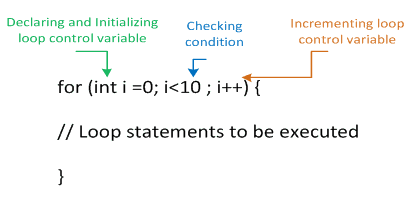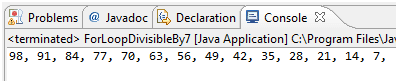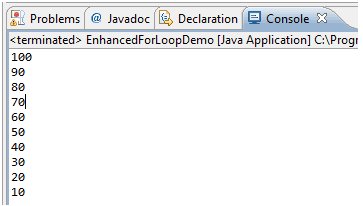# Java For Loop

## Description

A for loop is a special loop that is used when a definite number of loop iterations is required. Although a while loop can also be used to meet this requirement, the for loop provides you with a shorthand notation for this type of loop. When you use a for loop, you can indicate the starting value for the loop control variable, the test condition that controls loop entry, and the expression that alters the loop control variable—all in one convenient place. Below is the syntax of a conventional for loop.For loop will begin with the keyword for followed by a set of parentheses. Within the parentheses are three sections separated by exactly two semicolons. The three sections are usually used for the following:

• Initializing the loop control variable
• Testing the loop control variable
• Updating the loop control variable

Within the parentheses of the for statement shown in below program, the first section prior to the first semicolon declares a variable named count and initializes it to 1. The program executes this statement once, no matter how many times the body of the for loop executes. After initialization, program control passes to the middle, or test section, of the for statement. If the Boolean expression found there evaluates to true, the body of the for loop is entered. In the program, the counter is set to 1, so when counter < 11 is tested, it evaluates to true. The loop body prints the counter value. If you want multiple statements to execute within the loop, they have to be blocked within a pair of curly braces. After the loop body executes, the final one-third of the for loop executes, and the counter is increased to 2. Following the third section in the for statement, program control returns to the second section, where theh counter is compared to 11 a second time. Because the counter is still less than 11, the body executes: counter (now 2) prints, and then the third altering portion of the for loop executes again. The variable counter increases to 3 and the for loop continues.

Eventually, when the counter is not less than 11 (after 1 through 10 have printed), the for loop ends and the program continues with any statements that follow the for loop.

```for (int counter =1; counter<11 ; counter++) {System.out.println(counter);}
```

For loop is very useful in java programming and widely used in java programs. Let’s see few more samples for loop declaration.

• Initialization of more than one variable by placing commas between the separate statements, as in the following:
```for(g = 0, h = 1; g < 6; ++g)
```
• Checking of more than one condition using AND or OR operators, as in the following:
```  for(g = 0; g < 3 && h > 1; ++g, h--)
```
• Decrementing loop control variable and checking of some other condition, as in the following:
``` for(g = 5; g >= 1; --g)
```
• Altering more than one value, as in the following:
``` for(g = 0; g < 10; ++g, ++h, sum += g)
```
• You can leave one or more portions of a for loop empty, although the two semicolons are still required as placeholders. For example, if x has been initialized in a previous program statement, you might write the following:
``` for(; x < 10; ++x)
```

Let’s see below example which prints all the values divisible by 7 in the range of 1 to 100 in reverse order.

Java Code: Go to the editor

``````public class ForLoopDivisibleBy7 {
public static void main(String[] args) {
for(int i=100; i>0; --i)
{
if(i%7 == 0)
{
System.out.print(i);
System.out.print(", ");
}
}
}
}
```
```

Output:Enhanced for loop

The enhanced for loop allows you to cycle through an array/ Collection without specifying the starting and ending points for the loop control variable. The general form of the enhanced for loop version is shown here:

```for(type itr-var : collection) statement-block
```

Here, type specifies the type and itr-var specifies the name of an iteration variable that will receive the elements from a collection, one at a time, from beginning to end. Because the iteration variable receives values from the collection, the type must be the same as (or compatible with) the elements stored in the collection. Thus, when iterating over arrays, the type must be compatible with the base type of the array.

Below example shows the use of enhanced for loop.

Java Code: Go to the editor

``````public class EnhancedForLoopDemo {
public static void main(String[] args) {
int [] myArray = new int;
int i=0;
for(int k=100; k>0; k=k-10, i++)
{
myArray[i]=k;
}
//Enhanced for loop to print elements of array
for(int loopVal: myArray)
{
System.out.println(loopVal);
}
}
}
```
```

Output:As this output shows, the enhanced for loop style automatically cycles through an array in sequence from the lowest index to the highest.

Summary

• A for loop is a special loop that is used when a definite number of loop iterations is required.
• For loop have 3 sections, loop variable initialization, testing loop control variable, updating loop control variable.
• Enhanced for loop can be used to iterate through Array or collections.

Java Code Editor:

Previous: While and Do loop
Next: Java Branching Statements

﻿